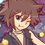# 2 Problems from math competition (OBEC IMSO) in Thailand.

I had a math comp. 3 days ago. And I can't do these problems. Stuck a lot. :<

1.) Triangle ABC given that AB = AC. Draw a line from B perpendicular to AC at point D. Draw another line from D perpendicular to BC at E. If BC = AB + AD, prove that BE = CD.

2.) Given a number $x$ with more than 1 digit. If we write it twice (such as x = 137, we write it 137137), we'll get a number that is divisible by $x^{2}$. Prove that the 2 first digits are 14......Note by Samuraiwarm Tsunayoshi
7 years, 4 months ago

This discussion board is a place to discuss our Daily Challenges and the math and science related to those challenges. Explanations are more than just a solution — they should explain the steps and thinking strategies that you used to obtain the solution. Comments should further the discussion of math and science.

When posting on Brilliant:

• Use the emojis to react to an explanation, whether you're congratulating a job well done , or just really confused .
• Ask specific questions about the challenge or the steps in somebody's explanation. Well-posed questions can add a lot to the discussion, but posting "I don't understand!" doesn't help anyone.
• Try to contribute something new to the discussion, whether it is an extension, generalization or other idea related to the challenge.

MarkdownAppears as
*italics* or _italics_ italics
**bold** or __bold__ bold
- bulleted- list
• bulleted
• list
1. numbered2. list
1. numbered
2. list
Note: you must add a full line of space before and after lists for them to show up correctly
paragraph 1paragraph 2

paragraph 1

paragraph 2

[example link](https://brilliant.org)example link
> This is a quote
This is a quote
    # I indented these lines
# 4 spaces, and now they show
# up as a code block.

print "hello world"
# I indented these lines
# 4 spaces, and now they show
# up as a code block.

print "hello world"
MathAppears as
Remember to wrap math in $$ ... $$ or $ ... $ to ensure proper formatting.
2 \times 3 $2 \times 3$
2^{34} $2^{34}$
a_{i-1} $a_{i-1}$
\frac{2}{3} $\frac{2}{3}$
\sqrt{2} $\sqrt{2}$
\sum_{i=1}^3 $\sum_{i=1}^3$
\sin \theta $\sin \theta$
\boxed{123} $\boxed{123}$

Sort by:

I just figured no. 2 out. XD

Given that $x^{2} | \overline{xx} \rightarrow x^{2} | x(10^{n}+1) \rightarrow x | (10^{n} + 1)$.

Factor $10^{n} + 1$ as $a\times b$ where $a, b$ are positive integers.

Since we know that both of them are odd numbers, but not divisible by 3 and 5 since $3, 5 \not| (10^{n} + 1)$.

So $a, b$ must be divisible by 7.

When we divide $10^{n} + 1$ by 7, it always starts with 1, 4 for $n \geq 2$. Hence, proven. ~~~

I always hate myself when I can't do it during the test but after the test. >:(

- 7 years, 4 months ago

Let angle $ACB$'s size be $x$.

Then angle $EDC$'s and angle $EDB$'s size will be $90-x$ and $x$, respectively.

From the properties, we will get that

$\bigtriangleup BDE \sim \bigtriangleup BCD$

Thus, $\frac{BE}{BD} = \frac{BD}{BC}$

$BE = \frac{BD^{2}}{BC} = \frac{AB^{2} - AD^{2}}{AB + BD}$ (Pythagorean Theorem)$= AB - AD = AC - AD$(Isosceles Triangle)$= DC$ $Q.E.D.$ ซ.ต.พ.

พิสูจน์สองข้อนี้ผมแป้กในห้องสอบทั้งคู่ครับ ไม่ทราบว่าได้เหรียญอะไรอ่อครับ

- 7 years, 2 months ago

ได้แค่เหรียญทองแดงเองคับ เสียไป 2 ข้อนี้ฟรีๆ เหมือนเส้นผมบังภูเขา T__T PS: ข้อแรกเพิ่งคิดได้ตอนนั่งคิดเล่นๆ ใช้เวลาแค่ 5 นาทีเสร็จ ==''

- 7 years, 2 months ago

ผมก็ได้แค่เหรียญทองแดงครับ เจอเรขานี้ Let it go แต่ข้อ Number Theory แสดงไปซุยๆครับแค่สองบรรทัดแรก 555

- 7 years, 2 months ago

เหมือนกันเลยครับ T__T แต่ข้อเรขา เรานั่งลุยถึกสมมติตัวแปร x แล้วไล่หาทุกด้าน แฮ่กๆๆๆ

- 7 years, 2 months ago

Hi Samuraiwarm!

Sorry, but I wasn't able to solve any of them. But could you please do me a favour?

Please send all the questions to me, I want to give all a try. My email address is sgsuper@yahoo.com, thanks.

- 7 years, 4 months ago